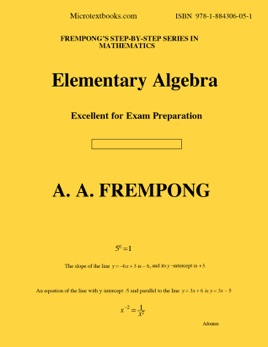• \$14.99

## Publisher Description

Elementary Algebra covers: Signed Number and Real Number Operations; Order of Operations and Evaluation of Expressions; Exponential Notation and Rules of Exponents; Polynomial addition, subtraction, multiplication, and division; Solving First Degree Equations;Word Problems; Factoring Polynomials; Solving quadratic equations by factoring & applications; Graphs, Slopes, Intercepts and Equations of Straight Lines; Solving Systems of Linear Equations and Word Problems; Radicals, square roots, addition & multiplication of radicals; Pythagorean Theorem and Applications; Areas and Perimeters; Algebraic Fractions (reduction, multiplication, division & addition); Solving Linear inequalities. Extra topics include Quadratic Equations,, Functions, Relations,, Functional Notation, Sketching Parabola, Solving Fractional or Rational Equations, Solving Radical Equations, Basic Review for Geometry